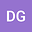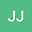••Oxygen ions are a major constituent of magnetospheric plasma, yet the role of oxygen in processes such as magnetic reconnection is poorly understood. Observations show that significant energized $O^+$ can be present in a magnetotail current sheet. A population of thermal $O^+$ only has a minor effect on magnetic reconnection. Despite this, published studies have so far only concentrated on the role of the low-energy thermal $O^+$. We present a study of magnetic reconnection in a thinning current sheet with energized $O^+$ present. Well-established, three-species, 2.5D Particle-In-Cell (PIC) kinetic simulations are used. Simulations of thermal $H^+$ and thermal $O^+$ validate our setup. We energize a thermal background $O^+$ based on published measurements. We apply a range of energization to the background $O^+$. We discuss the effects of energized $O^+$ on current sheet thinning and the onset and evolution of magnetic reconnection. Energized $O^+$ has a major impact on the onset and evolution of magnetic reconnection. Energized $O^+$ causes a two-regime onset response in a thinning current sheet. As energization increases in the lower-regime, reconnection develops at a single primary {X}-line, increases time-to-onset, and suppresses the rate of evolution. As energization continues to increase in the higher-regimes, reconnection develops at multiple {X}-lines, forming a stochastic plasmoid chain; decreases time-to-onset; and enhances evolution via a plasmoid instability. Energized $O^+$ drives a depletion of the background $H^+$ around the current sheet. As energization increases, the thinning begins to slow and eventually reverses, leading to disruption of the current sheet via a plasmoid instability.